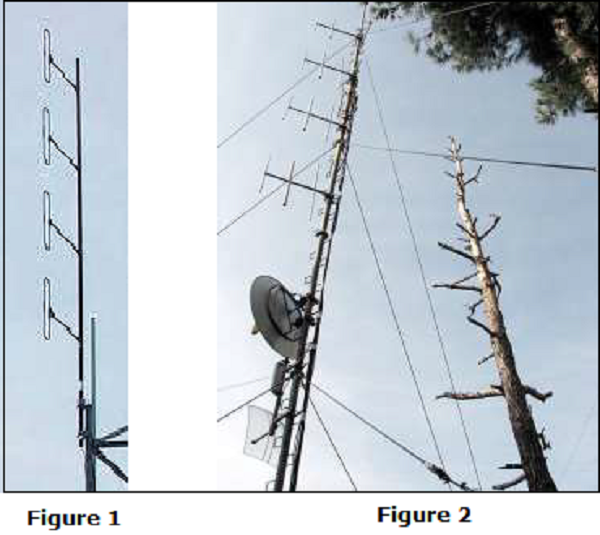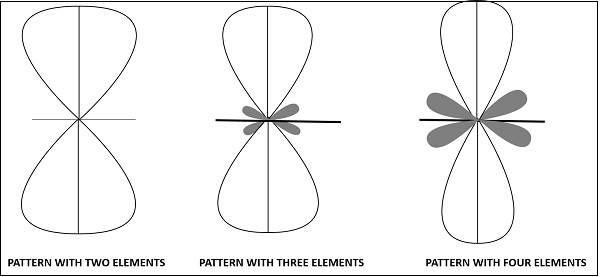# Antenna Theory - Collinear Array

A Collinear array consists of two or more half-wave dipoles, which are placed end to end. These antennas are placed on a common line or axis, being parallel or collinear.

The maximum radiation in these arrays is broad side and perpendicular to the line of array. These arrays are also called as broad cast or Omni-directional arrays.

### Frequency range

The frequency range in which the collinear array antennas operate is around 30 MHz to 3GHz which belong to the VHF and UHF bands.

## Construction of Array

These collinear arrays are uni-directional antennas having high gain. The main purpose of this array is to increase the power radiated and to provide high directional beam, by avoiding power loss in other directions.The above images show the pictures of collinear arrays. In figure 1, it is seen that collinear array is formed using folded dipoles, while in figure 2, the collinear array is formed by normal dipoles. Both types are half-wave dipoles used commonly.

The radiation pattern of these collinear arrays is similar to that of a single dipole, but the array pattern of increasing number of dipoles, makes the difference.The radiation pattern of collinear array when made using two elements, three elements and four elements respectively are shown in the figure given above.

The broad side array also has the same pattern, in which the direction of maximum radiation is perpendicular to the line of antenna.

The following are the advantages of collinear array antennas −

• Use of array reduces the broad ends and increases the directivity
• Minor lobes are minimised
• Wastage of power is reduced

The following are the disadvantages of collinear array antennas −

• Displacement of these antennas is a difficult task
• Used only in outdoor areas

### Applications

The following are the applications of collinear array antennas −

• Used for VHF and UHF bands
• Used in two-way communications
• Used also for broadcasting purposes